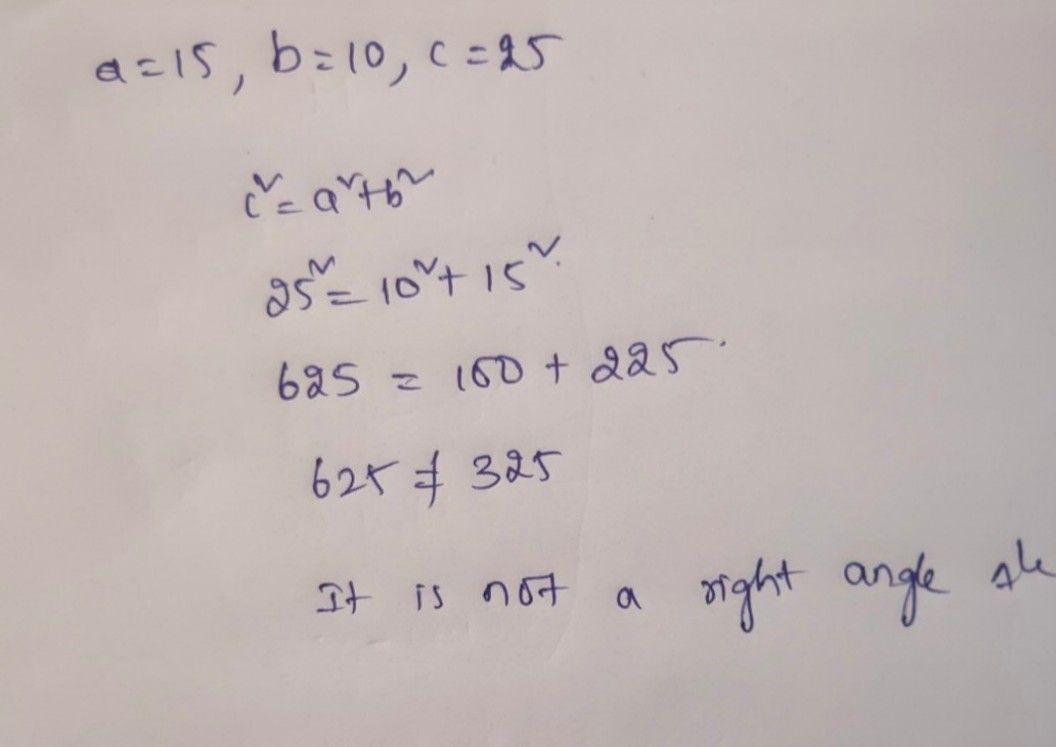Symbol
ProblemThe sides of a triangle are $15C∩$ $10C17$ and $A5\square i$ Determine if it is a right-angled triangle.
10th-13th grade
Trigonometry
Search count: 107
SolutionQanda teacher - anjali